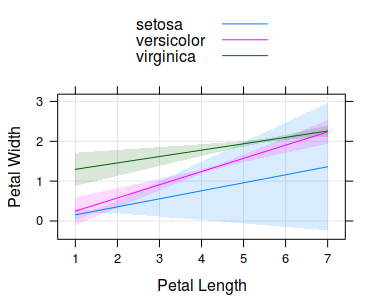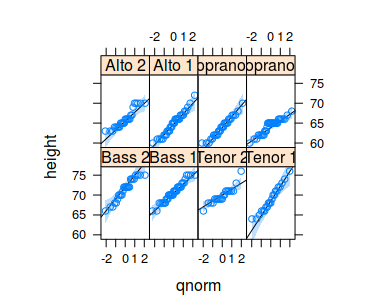# New panel functions

### tactile

#### 2018-04-23

tactile introduces new panel functions to the latticeverse.

## panel.ci(): confidence intervals

panel.ci() adds confidence bands around a line using arguments lower and upper. This is usually of interested after, for instance, having fitted a model and then made predictions using that model.

As an example, we will try to predict petal width from petal length and speices, using the iris dataset.

mod <- lm(Petal.Width ~ Petal.Length*Species, data = iris)
newdat <- expand.grid(Petal.Length = seq(1, 7, by = 0.1),
Species = c("setosa", "versicolor", "virginica"))
pred <- predict(mod, newdat, interval = "confidence")
dd <- cbind(newdat, pred)

Having predicted values across our grid, we now plot the predictions, including 95% confidence levels using the following lines.

library(tactile)
xyplot(fit ~ Petal.Length, groups = Species, data = dd,
prepanel = prepanel.ci, auto.key = list(lines = TRUE, points = FALSE),
ylab = "Petal Width",
xlab = "Petal Length",
lower = dd$lwr, upper = dd$upr,
type = "l",
panel = function(...) {
panel.ci(..., alpha = 0.15, grid = TRUE)
panel.xyplot(...)
})Example of using panel.ci.

Also note the use of the prepanel.ci() function that we provide the prepanel argument with so that the axis limits are set properly.

## panel.qqmathci(): confidence intervals for lattice::qqmath()

panel.qqmathci() is designed to be used together with lattice::qqmath() and lattice::panel.qqmathline() to provide confidence intervals for the theoretical quantities. A rather contrived example follows.

qqmath(~ height | voice.part, aspect = "xy", data = singer,
prepanel = prepanel.qqmathline,
distribution = qnorm,
ci = 0.9,
panel = function(x, ...) {
panel.qqmathci(x, ...)
panel.qqmathline(x, ...)
panel.qqmath(x, ...)
})Example of using panel.qqmathci.